# Inverse function facts for kids

Kids Encyclopedia Facts

An inverse function is a concept of mathematics. A function will calculate some output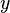$y$, given some input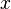$x$. This is usually written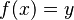$f(x) = y$. The inverse function does the reverse. Let's say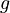$g$ is the inverse function of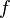$f$, then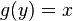$g(y) = x$. Or otherwise put,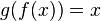$g(f(x)) = x$. An inverse function to$f$ is usually called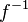$f^{-1}$. It is not to be confused with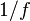$1/f$, which is a reciprocal function.

## Examples

If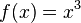$f(x) = x^3$ over real$x$, then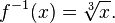$f^{-1}(x) = \sqrt{x}.$

To find the inverse function, swap the roles of$x$ and$y$ and solve for$y$. For example,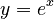$y=e^x$ would turn to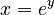$x=e^y$, and then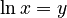$\ln x =y$. This shows that the inverse function of$y=e^x$ is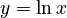$y=\ln x$.

Not all functions have inverse functions: for example, function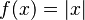$f(x) = |x|$ has none (because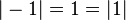$|-1| = 1 = |1|$, and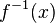$f^{-1}(x)$ cannot be both 1 and -1), but every binary relation has its own inverse relation.

In some cases, finding the inverse of a function can be very difficult to do.

## Images for kidsInverse function Facts for Kids. Kiddle Encyclopedia.